# How To Find Image Of Point In A Plane

A flat, two-dimensional [2d] surface, which extends infinitely is a plane. It is a 2d analogue of a point, a line and 3-dimensional space. A plane in 3d space is denoted by the equation

ax + by + cz + d=0,

where a, b and c are non-zero.

A point and a vector are perpendicular to the plane, which determines the plane in three-dimensional coordinate space.

## Steps to Find Image Of Point In A Plane

Consider the 2 points P and Q. Let π be a plane such that

• There exists a perpendicular line PQ to the plane π.
• The midpoint of PQ is on the plane π. Then, the image of the point is either of the points to one another in the plane π. The procedure to find the image of a point in a given plane is as follows:
• The equations of the normal to the given plane and the line passing through the point P are written as $\frac{x-x_1}{a} = \frac{y-y_1}{b} = \frac{z-z_1}{c}$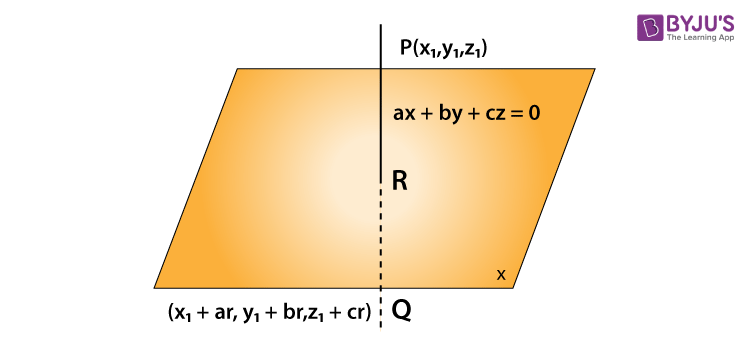• The coordinates of the image Q are (x1+ ar, y1 + br, z1 + cr).
• The coordinates of the mid-point R of PQ are found.
• The value of r is obtained by substituting the coordinates of R in the plane equation.
• Finally, the r-value is put in the coordinates of Q.

## Solved Problems

Example 1: Find the image of the point (0, 0, 0) in the plane 3x + 4y – 6z + 1 = 0.

Solution: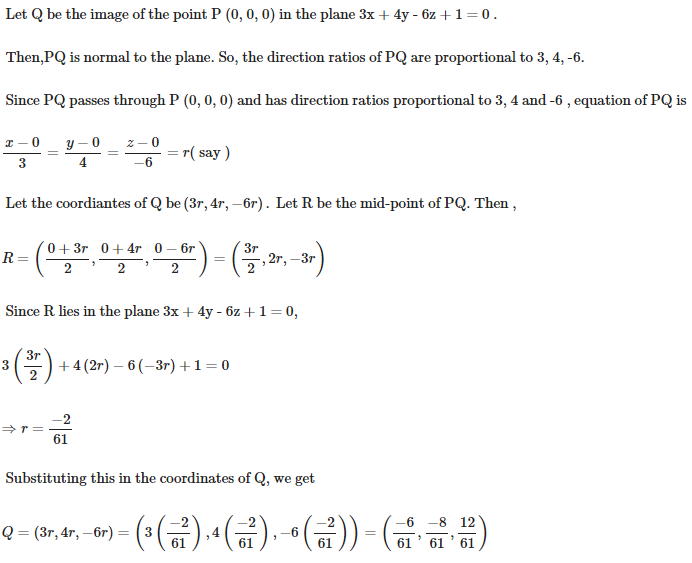Example 2: Find the reflection of the point (1, 2, -1) in the plane 3x – 5y +4z = 5.

Solution: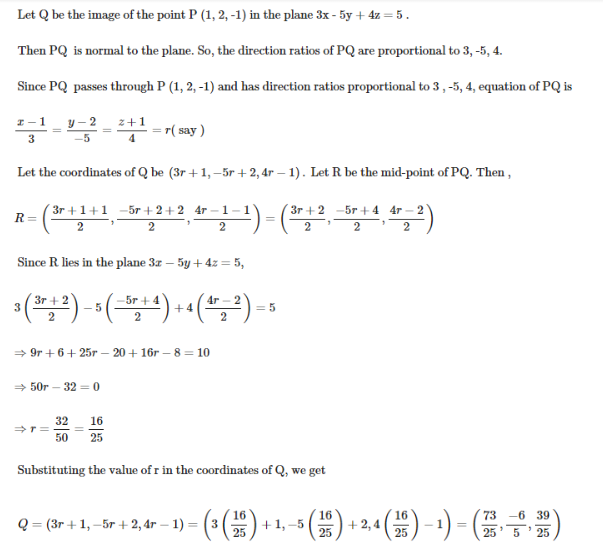Example 3: Find the image of the point (1, 2, 3) in the plane x + 2y + 4z – 38 =0.

Solution: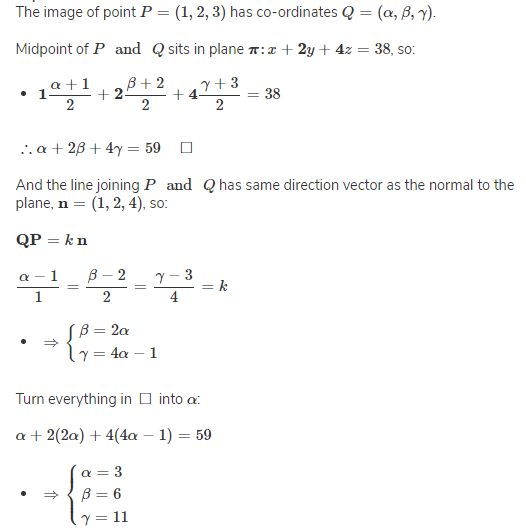Example 4: The image of the point P (1, 3, 4) in the plane 2x − y + z + 3 = 0, is __________.

Solution:

The equation of a line through the point P (1, 3, 4) and perpendicular to the plane 2x − y + z + 3 = 0 is

$\frac{x-1}{2} = \frac{y-3}{-1} = \frac{z-4}{1}$ = k (say)

A general point on this line is (2k + 1, −k + 3, k + 4).

For some value of k let N (2k + 1, −k + 3, k + 4) be a point of the line lying on the plane. Then,

2 (2k + 1) − (−k + 3) + (k + 4) + 3 = 0

⇒ 6k = −6

⇒ k = −1.

∴ Coordinates of N are (−2 + 1, 1 + 4, −1 + 4, −1 + 4), i.e., N (−1, 4, 3).

Let Q (α, β, γ) be the image of P in the given plane. Then,

[1 + α] /  = −1, [3 + β] /  = 4 and [4 + γ] / .

So, α = −3, β = 5 and γ = 2k.

Hence, the required image of P (1, 3, 4) in the given plane is Q (−3, 5, 2).

Example 5: Find the image of point P (1, 2, 3) with respect to plane x + y + z.

Solution: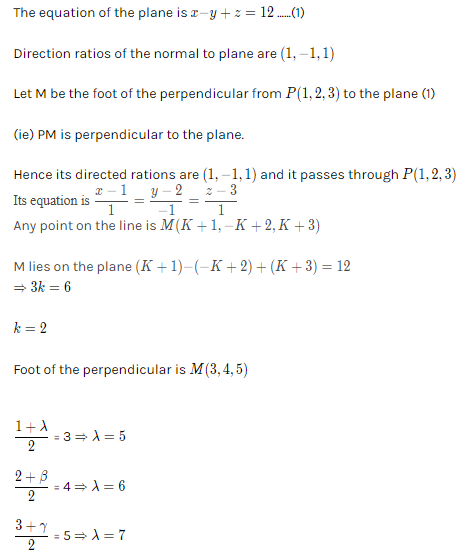Example 6: Find the image of the point (5, 2, 6) in the plane x + y + z = 9.

Solution:

Let I be the given Point and I’ be the image.

The plane equation is x + y + z = 9 —— (1).

Direction ratios of the normal to the plane are (1, 1, 1).

Let P be the foot of the perpendicular from I (5, 2, 6) to the plane (1). PI is perpendicular to the plane.

So, the direction ratios are (1, 1, 1) and it passes through I (5, 2, 6).

Its equation is $\frac{x-5}{1}+\frac{y-2}{1}+\frac{z-6}{1}=K$

Any point on the line is P (K + 5, K + 2, K + 6)

Since P lies on the plane, $(K+5)+(K+2)+(K+6)=9 \Rightarrow K = \frac{-4}{3} ​$

Foot of the perpendicular is P $(\frac{11}{3},\frac{2}{3},\frac{14}{3}) ​$

P is the mid point of I.

$\frac{5+ \lambda }{2}=\frac{11}{3} \Rightarrow \lambda = \frac{7}{3}\\ \frac{2+ \beta }{2}=\frac{2}{3} \Rightarrow \beta = \frac{-2}{3}\\ \frac{6+ \gamma }{2}=\frac{14}{3} \Rightarrow \gamma = \frac{10}{3}​$

So, I’ = $(\frac{7}{3},\frac{-2}{3},\frac{10}{3}) ​$

Example 7: If the image of the point B (1, 2, -3) relative to the plane P is A (-3, 6, 4) then find the equation of the plane P.

Solution:

Let the equation of the plane be ax + by + cz = k.

As the two points are images with respect to the plane.

The mid point lies on the plane = $(\frac{1+(-3)}{2},\frac{2+6}{2},\frac{-3+4}{2}) ​$

Mid point = $(-1,4,\frac{1}{2}) ​$

Substitute in the plane equation,

⇒  −a + 4b + 0.5c = k        —- ( 1 ) [a, b, c is the slope of the line joining BA]

∴  a = −3 −1 = −4; b = 6 − 2 = 4; c = 4 − (−3) = 7

⇒  −(−4) + 4 (4) + 0.5 (7) = k

∴  k = 23.5

∴  The equation of plane P = 2 (−4x + 4y + 7z) = 2 (23.5)

∴   The required equation is 8x − 8y − 14z + 47 = 0